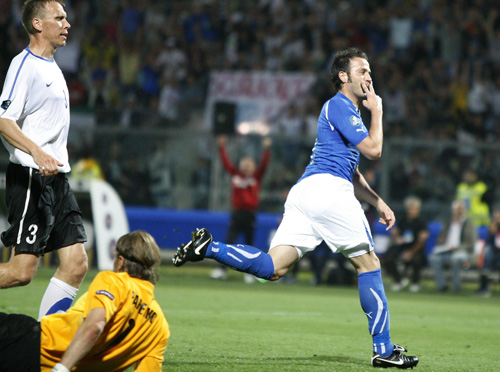# イタリア代表EURO2012予選 6/3 20:45-イタリア 3 - 0 エストニア

#### イタリアが３ゴール快勝！　指揮官「まだまだよくなる」

ラノッキアはフル出場、パッツィーニは64分から出場＋チーム３点目を決めています。

グループC チーム 勝点 勝+分+敗 得失差
1.イタリア 16 6=5+1+0 14-1=+13
2. スロべニア 11 7=3+2+2 9-4=+5
3.セルビア 8 6=2+2+2 8-9=-1
4. エストニア 7 6=2+1+3 7-9=-2
5. 北アイルランド 6 5=1+3+1 3-3=0
6. フェロー諸島 1 6=0+1+5 3-18=-15

セルビア/北アイルランドは今節は試合なし。（セルビアは韓国でテストマッチ